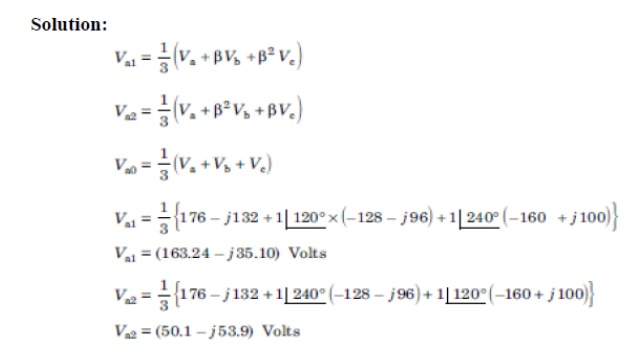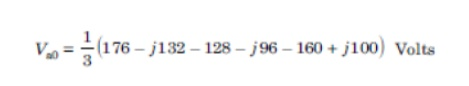Home | | Power System Analysis | Sequence Impedances Sequence Networks

# Sequence Impedances Sequence Networks

The sequence impedances are the impedances offered by the power system components or elements to +ve, -ve and zero sequence current.

SEQUENCE IMPEDANCES SEQUENCE NETWORKS

The sequence impedances are the impedances offered by the power system components or elements to +ve, -ve and zero sequence current.

The single phase equivalent circuit of power system consisting of impedances to current of any one sequence only is called sequence network.

The phase voltage across a certain load are given asCompute positive, negative and zero sequence component of voltageA balanced delta connected load is connected to a three phase system and supplied to it is a current of 15 amps. If the fuse is one of the lines melts, compute the symmetrical components of line currents.Draw zero sequence network of the power system as shown in fig.Draw zero sequence network of the power system as shown in fig.Draw zero sequence network of the power system as shown in fig. Data are given below.Study Material, Lecturing Notes, Assignment, Reference, Wiki description explanation, brief detail
Power System Analysis : Symmetrical Components And Unbalanced Fault Analysis : Sequence Impedances Sequence Networks |